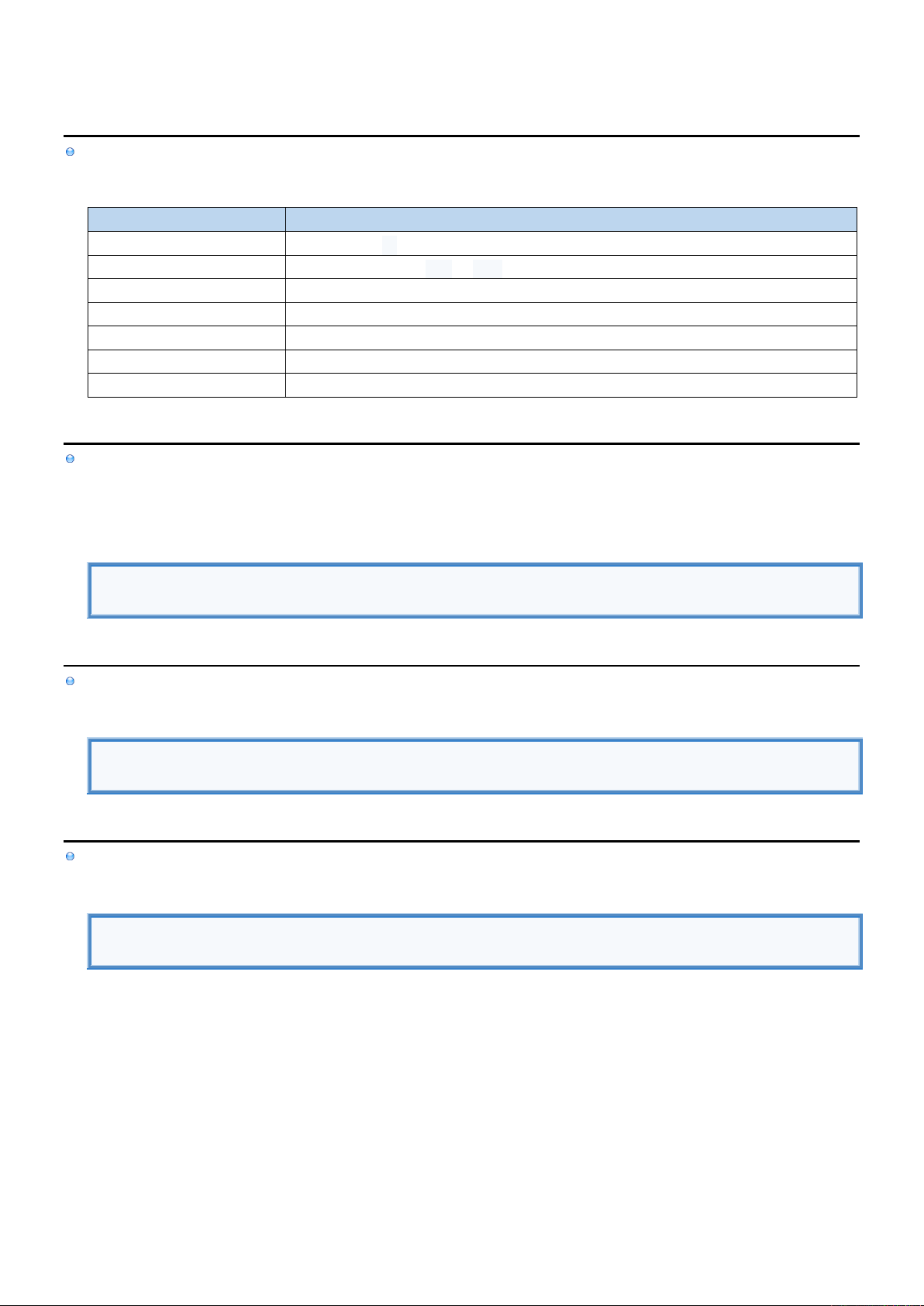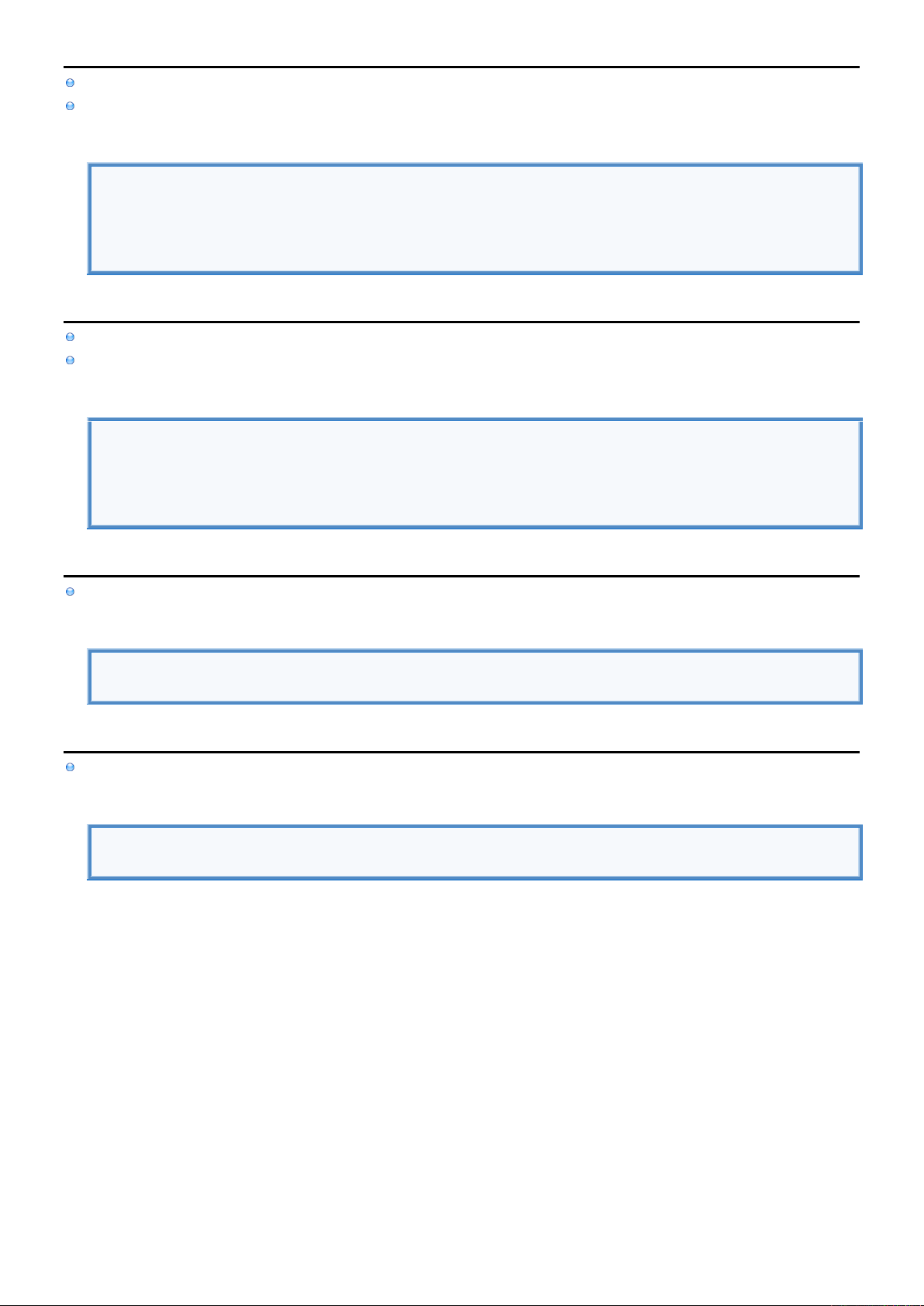2
2
.
.
2
2
.
.
2
2
S
S
c
c
a
a
l
l
a
a
r
r
s
s
I
I
n
n
f
f
o
o
Scalar Data Types are implemented as Structures and can only store single value.
Scalar Data Types
TYPE
DESCRIPTION
Nil
Can only have nil value, It can only be assigned to optional.
Bool
Can only have logical true or false values.
String
Represents sequence of characters treated as a continuous group
Int
Different signed integer data types can store signed integers of various sizes
UInt
Different unsigned integer data types can store unsigned integers of various sizes
Float
Represents real number as 32-bit floating-point number.
Double
Represents real number as 64-bit floating-point number.
N
N
i
i
l
l
Nil Data Type can only have nil value and can only be assigned to Optional either by
explicitly setting Optional to nil using Nil Literal
declaring Optional without assigning value to it in which case it is initialized to nil
Nil Data Type
var age : Int? //Optional variable is initialized to nil data type.
var weight : Int? = nil //Optional variable is set to nil data type using nil literal.
B
B
o
o
o
o
l
l
Bool Data Type can only have two possible values logical true or false and can be created using Boolean Literal.
Bool Data Type
var access1 = true
var access2 : Bool = false
S
S
t
t
r
r
i
i
n
n
g
g
String Data Type represents sequence of characters and can be created using String Literal.
String Data Type
var name1 = "John" //Implicit type definition.
var name2 : String = "John" //Explicit type definition.I
I
n
n
t
t
Int Data Type can store signed integers of various sizes and can be created using Integer Literal
Int is alias for Int32 or Int64 depending if the platform is 32-bit or 64-bit respectively.
Int Data Type
var a : Int = -50 // Alias for Int32 or Int64 if platform is 32-bit or 64-bit respectively
var b : Int8 = +50 // Signed integer with 8 bits
var c : Int16 = 50 // Signed integer with 16 bits
var d : Int32 = 50 // Signed integer with 32 bits
var e : Int64 = 50 // Signed integer with 64 bits
U
U
I
I
n
n
t
t
UInt Data Type can store unsigned (positive) integers of various sizes and can be created using Integer Literal
UInt is alias for UInt32 or UInt64 depending if the platform is 32-bit or 64-bit respectively.
UInt Data Type
var a : UInt = 50 // Alias for UInt32 or UInt64 if platform is 32-bit or 64-bit respectively
var b : UInt8 = +50 // Unsigned integer with 8 bits
var c : UInt16 = 50 // Unsigned integer with 16 bits
var d : UInt32 = 50 // Unsigned integer with 32 bits
var e : UInt64 = 50 // Unsigned integer with 64 bits
F
F
l
l
o
o
a
a
t
t
Float Data Type represents real number as 32-bit floating-point number and can be created using Float Literal.
Float Data Type
var a : Float = 50.36
var b : Float = 0.536E+2
D
D
o
o
u
u
b
b
l
l
e
e
Double Data Type represents real number as 64-bit floating-point number and can be created using Float Literal.
Double Data Type
var a:Double = 50.36
var b:Double = 0.536E+2